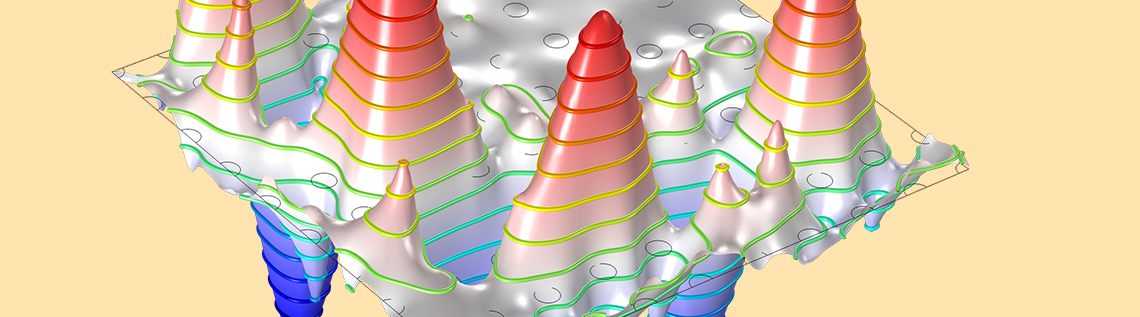# Engineering the Flow of Light Using Photonic Crystals

July 25, 2019

In 1980, Eli Yablonovitch from Bell Communication Research pondered how to reduce losses in semiconductor lasers in a specific frequency range. He sliced periodic circular holes in a transparent medium and observed that it didn’t allow the frequency range causing the losses to pass through. Yablonovitch found that these structures work similarly to semiconductors with conduction and valence bands and named them photonic crystals (with Sajeev John from Princeton University). Let’s discuss three examples in which photonic crystals control light.

### Bending Light with a Photonic Crystal

When GaAs pillars are arranged in a periodic manner, as shown in the figure below, the device has the ability to bend light with an angle (90° in this case) and also act as a filter for a band of frequencies (also called a photonic band gap).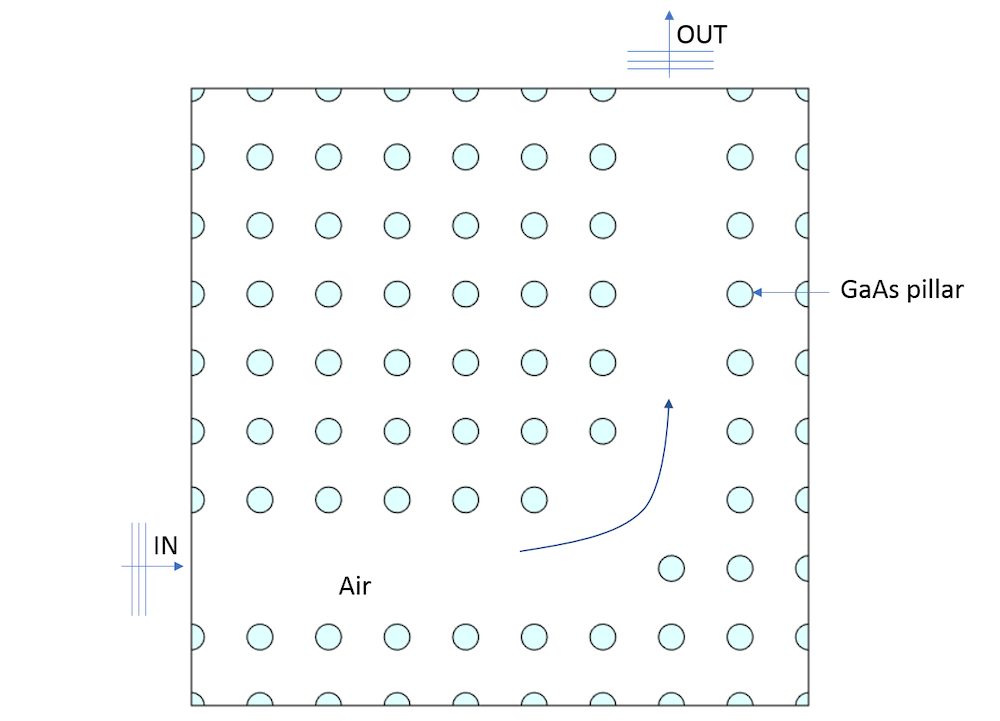Schematic of a photonic crystal.

To model this photonic crystal in the COMSOL Multiphysics® software and add-on Wave Optics Module, the transverse electric (TE) wave (polarized in the z direction) is made to propagate through the left boundary using the Scattering boundary condition and an amplitude of 1 V/m. The rest of the boundaries are assigned Scattering boundary conditions with no incident fields. When we run the model with a sweep of different wavelengths for the incident light source, we get a graph of the transmittance and reflections, as shown below.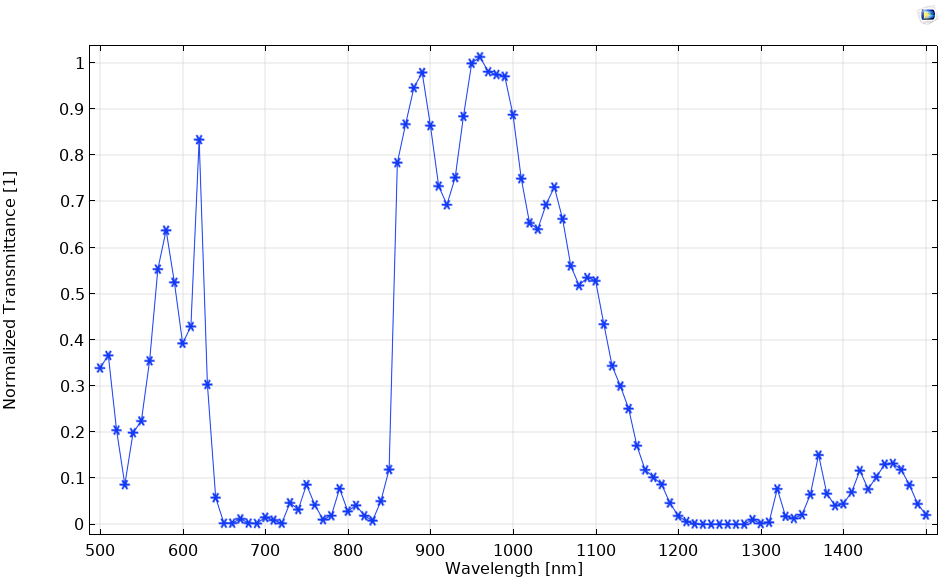Normalized transmittance of the photonic crystal.

Left: Passband at a 1000-nm wavelength. Right: Stopband at a 700-nm wavelength.

### Modeling a Photonic Crystal Fiber

A step-index fiber guides light through the high refractive index of the core, while a photonic crystal fiber (PCF) is made up of microstructured optical fibers that guide light either through index guiding or band gap confinement. In this blog post, we focus on index-guided PCF, where the core of the PCF is made of cladding material and is surrounded by air-filled holes. We assume the air hole’s radius as 0.3*pitch, where the pitch is the distance between adjacent holes.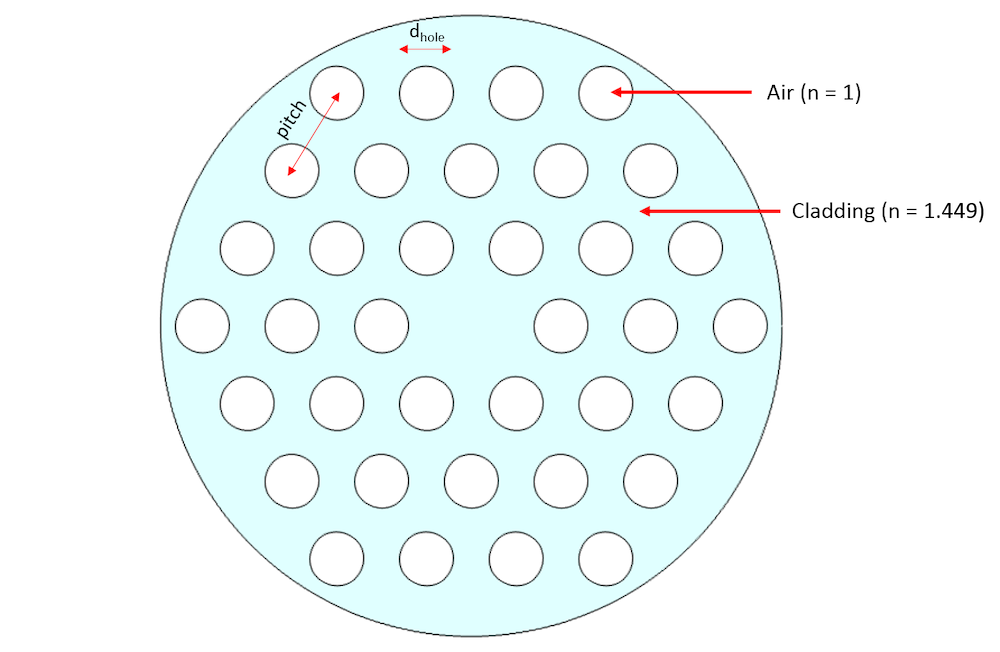Schematic of index-guided photonic crystal.

To plot the dispersion diagram (the effective refractive index vs. the normalized wavelength), we perform a mode analysis along with a parametric sweep over the hole radius from 0.23 um to 4.69 um. To detect the fundamental and higher-order modes, the number of modes to search is increased to 50. The challenge then becomes correctly identifying the fundamental and higher-order modes from a total of 50 identified modes. One approach to identify these modes is to perform an integration in the core region for different effective mode indices (or effective refractive indices).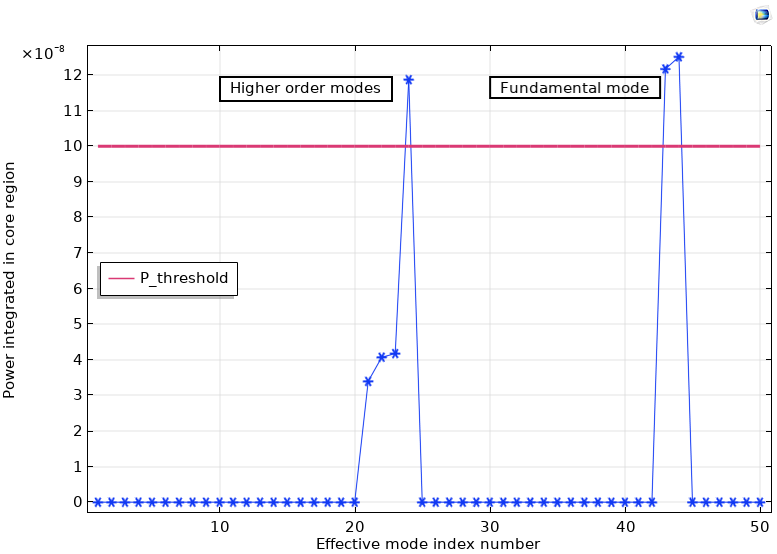Power integrated in the core region vs. the effective mode index number for a 4.65-um hole radius and 15.5-um pitch.

There are two approaches to filter out unnecessary modes and capture only the meaningful fundamental and higher-order modes:

1. Apply a filter to the power; for example, the required effective refractive index is then `ewfd.neff*(intop1(ewfd.Poavz)>P_threshold)`, where ` P_threshold` is the power that will eliminate the unnecessary modes
2. Observe the effective mode index number for the fundamental and higher-order modes and if it is getting repeated

In this case, we observe that the fundamental mode is repeated between effective mode index numbers 40 and 45, and higher-order modes were repeated between effective mode index numbers 20 and 25. The dispersion diagram is plotted by included both of these filters to remove the unnecessary modes. The dispersion diagram matches well with Figure 4, Chapter 9 in Ref. 1.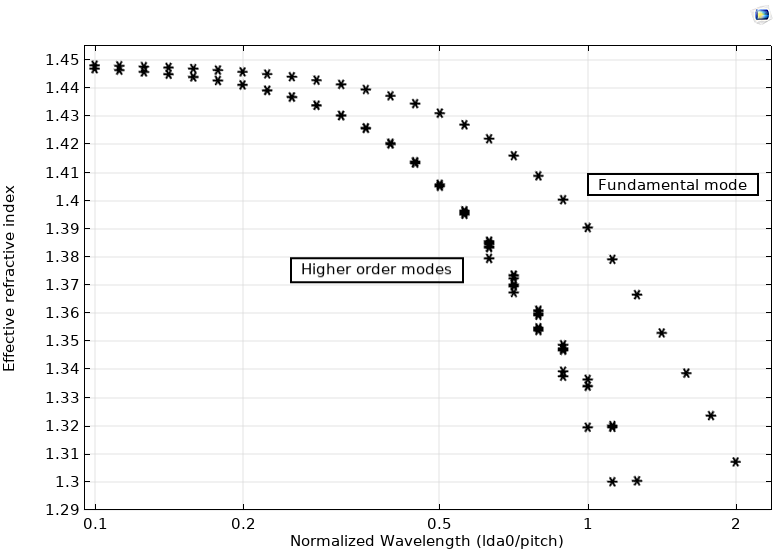Dispersion diagram: effective refractive index vs. normalized wavelength (lda0/pitch).

### Analyzing a Photonic Band Gap

An alternate approach to modeling the band gap is to formulate an eigenvalue problem, as shown in the Band Gap Analysis of a Photonic Crystal model. In this case, a periodic arrangement of GaAs pillars is modeled in which the pillars are placed equidistant from each other. Instead of modeling an array of GaAs pillars, as we did in the first example, we model only a single unit cell and apply a Floquet periodic boundary condition, as shown below.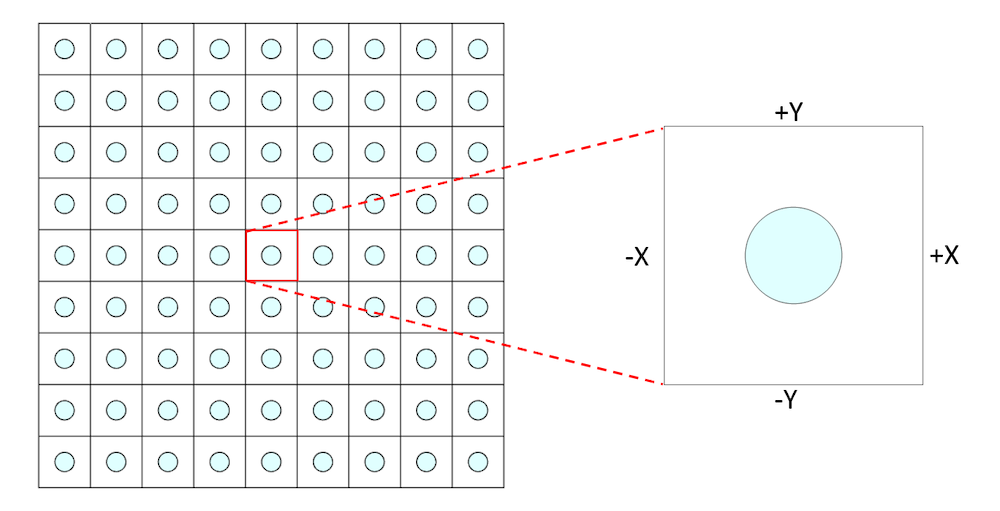Array to unit approximation with the Periodic boundary condition applied at +/- X and +/-Y.

An auxiliary sweep is performed on the wave vector k from 0 to 0.5 to evaluate the dispersion relation in the (1,1) direction. Additionally, the refractive index of the GaAs material is considered frequency dependent. As can be seen below from the dispersion relationship, there is no EM wave propagation surviving in the (1,1) direction between bands 3 and 4, also known as the band gap of the photonic crystal.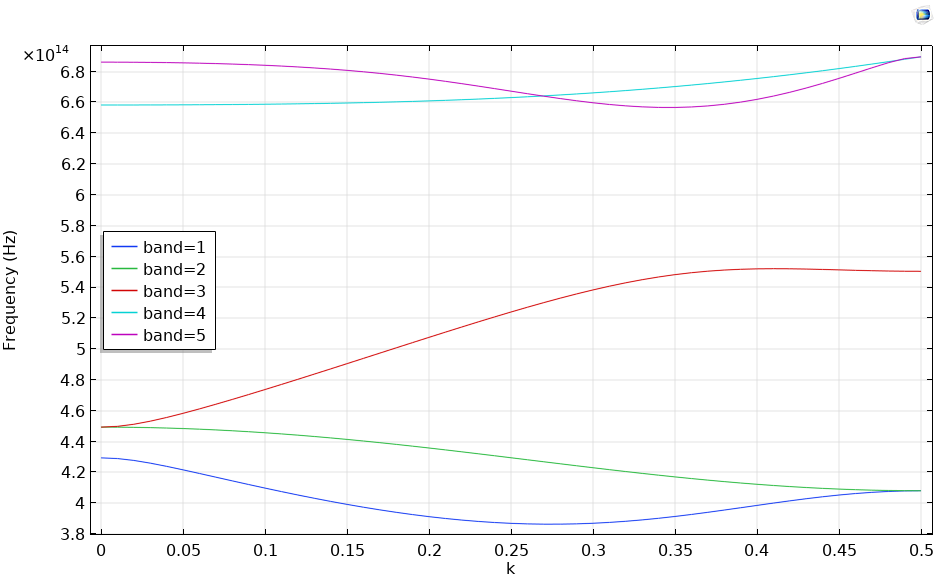Dispersion relation when the wave vector is varied from 0 to 0.5 in the (1,1) direction.

### Final Thoughts on Photonic Crystals

There are different approaches to modeling photonic crystal devices, whether you perform a parametric sweep of different frequencies, analyze the modes, or solve for the eigenvalues. Photonic crystals can work as filters and tools to engineer the path of light, which is especially helpful while designing photonic integrated circuits. Additionally, when performing mode analysis, integrating the power at the core region and filtering the certain mode index number can help to delineate the fundamental and higher-order modes from other unnecessary modes. Finally, a unit cell model, along with Floquet periodic boundary conditions, can be modeled to perform band gap analysis.

### Next Steps

• Try the tutorial models featured in this blog post:

### Reference

1. J.D. Joannopoulus, R.D. Meade, and J.N. Winn, Photonic Crystals (Modeling the Flow of Light), Princeton University Press, 2008.

#### Categories##### 丹红 周
July 29, 2019##### Shweta Mittal
December 17, 2019

how we can plot this dispersion and effective index curve in comsol?##### Uttam Pal
December 19, 2019 COMSOL Employee

Hi Shweta, I would suggest you go through the model https://www.comsol.com/model/photonic-crystal-fiber-blog-model-75371 , where we created a dispersion curve with effective refractive index.##### Lazyalways
February 25, 2020

How to calculate the transmittance graph?##### Uttam Pal
February 27, 2020 COMSOL Employee

Hi Lazyalways, you can evaluate the transmittance graph using either the scattering variables S21 or the prebuild variable ewbe.Tport_2, as you can see in the optical ring resonator model: https://www.comsol.com/model/22221

EXPLORE COMSOL BLOG